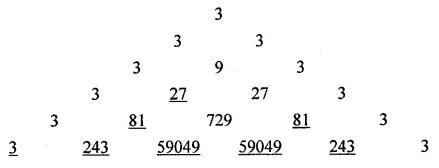# ML Aggarwal Class 8 Solutions for ICSE Maths Chapter 5 Playing with Numbers Ex 5.2

## ML Aggarwal Class 8 Solutions for ICSE Maths Chapter 5 Playing with Numbers Ex 5.2

Find the values of the letters in each of the following and give reasons for the steps involved (1 to 11):
Question 1.Solution:Question 2.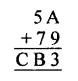Solution: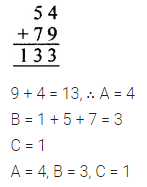Question 3.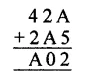Solution: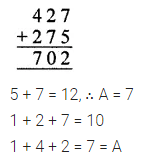Question 4.Solution: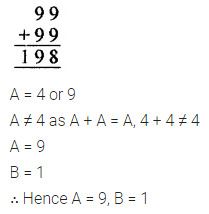Question 5.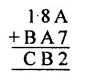Solution:Question 6.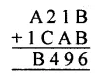Solution: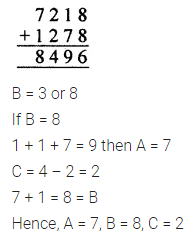Question 7.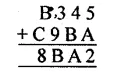Solution:Question 8.Solution: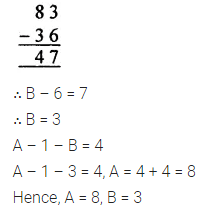Question 9.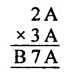Solution:Question 10.Solution: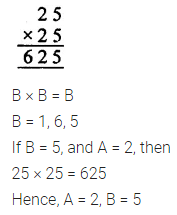Question 11.Solution: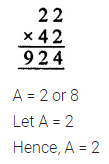Question 12.
Fill in the numbers from 1 to 6 (without repetition) so that each side of the magic triangle adds up to 12.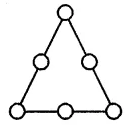Solution: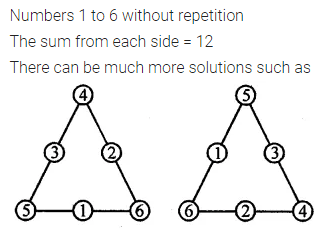Question 13.
Complete the magic square given alongside using number 0, 1, 2, 3, ……., 15 (only once), so that sum along each row, column and diagonal is 30.Solution: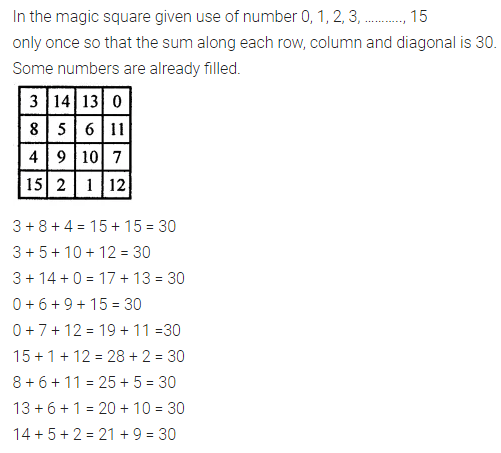Question 14.
Fill in the blanks to complete the following number triangle:Solution: